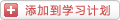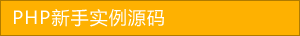﻿ PHP如何计算两个时间段交集的天数？ - php日期 - PHP粉丝网

# PHP如何计算两个时间段交集的天数？

##### 发布:smiling　来源: PHP粉丝网 　添加日期：2022-06-13 08:45:23　浏览: 评论：0

1. /**
2.
3.  * 计算两个时间段之间交集的天数
4.
5.  * @param \$startDate1 开始日期1
6.
7.  * @param \$endDate1 结束日期1
8.
9.  * @param \$startDate2 开始日期2
10.
11.  * @param \$endDate2 结束日期2
12.
13.  */
14.
15. public function share_date_days(\$startDate1\$endDate1\$startDate2\$endDate2
16.
17.
18.   \$days = 0;
19.
20.   \$startDate1 = strtotime(\$startDate1);// 开始日期1
21.
22.   \$endDate1 = strtotime(\$endDate1);// 结束日期1
23.
24.   \$startDate2 = strtotime(\$startDate2);// 开始日期2
25.
26.   \$endDate2 = strtotime(\$endDate2);// 结束日期2
27.
28.
29.
30.   /** ------------ 临界值换算 ------start------ */
31.
32.   // 如果日期1的结束日期小于日期二的开始日期，则返回0
33.
34.   if(\$endDate1 < \$startDate2){
35.
36.     \$days = 0;
37.
38.   }
39.
40.   // 如果日期1的开始日期小于日期二的结束日期，则返回0
41.
42.   if(\$startDate1 > \$endDate2){
43.
44.     \$days = 0;
45.
46.   }
47.
48.   // 如果日期1的结束日期等于日期2的开始日期，则返回1
49.
50.   if(\$endDate1 == \$startDate2){
51.
52.     \$days = 1;
53.
54.   }
55.
56.   // 如果日期1的开始日期等于日期2的结束日期，则返回1
57.
58.   if(\$startDate2 == \$endDate1){
59.
60.     \$days = 1;
61.
62.   }
63.
64.   /** ------------ 临界值换算 ------end------ */
65.
66.
67.
68.   /** ------------ 交集换算 ------start------ */
69.
70.   // 如果开始日期1小于开始日期2，且开始日期2小于结束小于结束日期1
71.
72.   if(\$startDate1 < \$startDate2 && \$endDate1 > \$startDate2){
73.
74.     // 如果结束日期1小于或者等于结束日期2
75.
76.     if(\$endDate1 <= \$endDate2){
77.
78.       \$days = \$this->diffBetweenTwoDays(\$startDate2\$endDate1) + 1;
79.
80.     }
81.
82.     // 如果结束日期1大于结束日期2
83.
84.     if(\$endDate1 > \$endDate2){
85.
86.       \$days = \$this->diffBetweenTwoDays(\$startDate2\$endDate2) + 1;
87.
88.     }
89.
90.   }
91.
92.
93.
94.   // 如果开始日期1大于开始日期2，且开始日期1小于结束日期2
95.
96.   if(\$startDate1 > \$startDate2 && \$startDate1 < \$endDate2){
97.
98.     // 如果结束日期1小于等于结束日期2
99.
100.     if(\$endDate1 <= \$endDate2){
101.
102.       \$days = \$this->diffBetweenTwoDays(\$startDate1\$endDate2) + 1;
103.
104.     }
105.
106.     // 如果结束日期1大于结束日期2
107.
108.     if(\$endDate1 > \$endDate2){
109.
110.       \$days = \$this->diffBetweenTwoDays(\$startDate1\$endDate2) + 1;
111.
112.     }
113.
114.   }
115.
116.   // 开始日期1等于开始日期2
117.
118.   if(\$startDate1 == \$startDate2){
119.
120.     // 结束日期1小于等于结束日期2
121.
122.     if(\$endDate1 <= \$endDate2){
123.
124.       \$days = \$this->diffBetweenTwoDays(\$startDate1\$endDate1) + 1;
125.
126.     }
127.
128.     // 结束日期1大于结束日期2
129.
130.     if(\$endDate1 > \$endDate2){
131.
132.       \$days = \$this->diffBetweenTwoDays(\$startDate1\$endDate2) + 1;
133.
134.     }
135.
136.   }
137.
138.   // 结束日期1等于结束日期2
139.
140.   if(\$endDate1 == \$endDate2){
141.
142.     // 开始日期1小于等于开始日期2
143.
144.     if(\$startDate1 <= \$startDate2){
145.
146.       \$days = \$this->diffBetweenTwoDays(\$startDate2\$endDate1) + 1;
147.
148.     }
149.
150.     // 开始日期1大于开始日期2
151.
152.     if(\$startDate1 > \$startDate2){
153.
154.       \$days = \$this->diffBetweenTwoDays(\$startDate1\$endDate1) + 1;
155.
156.     }
157.
158.
159.
160.   }
161.
162.   // 时间段1在时间段2内
163.
164.   if(\$startDate1 >= \$startDate2 && \$endDate1 <= \$endDate2){
165.
166.     \$days = \$this->diffBetweenTwoDays(\$startDate1\$endDate1) + 1;
167.
168.   }
169.
170.   // 时间段1包含时间段2
171.
172.   if(\$startDate1 < \$startDate2 && \$endDate1 > \$endDate2){
173.
174.     \$days = \$this->diffBetweenTwoDays(\$startDate2\$endDate2) + 1;
175.
176.   }
177.
178.   /** ------------ 交集换算 ------end------ */
179.
180.
181.
182.   return \$days
183.
184.
185.
186. /**
187.
188.  * 求两个日期之间相差的天数
189.
190.  * (针对1970年1月1日之后，求之前可以采用泰勒公式)
191.
192.  * @param string \$day1
193.
194.  * @param string \$day2
195.
196.  * @return number
197.
198.  */
199.
200. function diffBetweenTwoDays(\$day1\$day2
201.
202.
203.   if (\$day1 < \$day2) {
204.
205.     \$tmp = \$day2
206.
207.     \$day2 = \$day1
208.
209.     \$day1 = \$tmp
210.
211.   }
212.
213.   return (\$day1 - \$day2) / 86400;
214.
215. }

### 分享到： document.write('<a href="http://v.t.sina.com.cn/share/share.php?url='+encodeURIComponent(location.href)+'&appkey=3172366919&title='+encodeURIComponent('PHP如何计算两个时间段交集的天数？')+'" title="分享到新浪微博" class="t1" target="_blank">&nbsp;</a>'); document.write('<a href="http://www.douban.com/recommend/?url='+encodeURIComponent(location.href)+'&title='+encodeURIComponent('PHP如何计算两个时间段交集的天数？')+'" title="分享到豆瓣" class="t2" target="_blank">&nbsp;</a>'); document.write('<a href="http://share.renren.com/share/buttonshare.do?link='+encodeURIComponent(location.href)+'&title='+encodeURIComponent('PHP如何计算两个时间段交集的天数？')+'" title="分享到人人" class="t3" target="_blank">&nbsp;</a>'); document.write('<a href="http://www.kaixin001.com/repaste/share.php?rtitle='+encodeURIComponent('PHP如何计算两个时间段交集的天数？')+'&rurl='+encodeURIComponent(location.href)+'&rcontent=" title="分享到开心网" class="t4" target="_blank">&nbsp;</a>'); document.write('<a href="http://sns.qzone.qq.com/cgi-bin/qzshare/cgi_qzshare_onekey?url='+encodeURIComponent(location.href)+'" title="分享到QQ空间" class="t5" target="_blank">&nbsp;</a>');